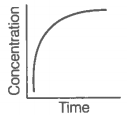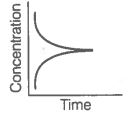42. Match the following graphical variation with their description.

A

B

A.1. Variation in product concentration with time.

B.2. Reaction at equilibrium

C.3. Variation in reactant concentration with time.

A.$\to$(2) B.$\to$(5) C.$\to$(3) D.$\to$(4)
As conjugate base$\to$Base+H+